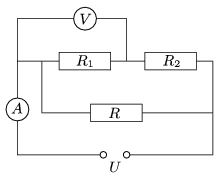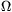Mathematical and Physical Journal
for High Schools
Issued by the MATFUND Foundation
 Already signed up? New to KöMaL?

#Problem P. 4447. (May 2012)

P. 4447. What are the resistances of the resistors R1 and R2, shown in the figure, if the voltage supply is 40 V, and the power dissipated on resistor R is 80 W, the readings on the ammeter and on the voltmeter are 3 A and 30 V, respectively.(4 pont)

Deadline expired on June 11, 2012.

Sorry, the solution is available only in Hungarian. Google translation

Megoldásvázlat. R1=30és R2=10.

### Statistics:

 112 students sent a solution. 4 points: 110 students. 2 points: 1 student. 1 point: 1 student.

Problems in Physics of KöMaL, May 2012